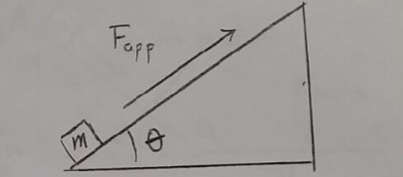Problem: Consider the arrangement shown in the figure. A box of mass m = 5.00 kg is pushed up a slope of inclination angle θ = 25° by an applied force Fapp. The coefficients of static and kinetic friction between the box and the inclined plane are μs = 0.30 and μk = 0.20 respectively. Make a force diagram showing all the forces acting on the box. Your diagram should have four forces in it. With the help of your diagram, determine the magnitude of the normal force acting on the box.

FREE Expert Solution
92% (134 ratings)
Problem Details

Consider the arrangement shown in the figure. A box of mass m = 5.00 kg is pushed up a slope of inclination angle θ = 25° by an applied force Fapp. The coefficients of static and kinetic friction between the box and the inclined plane are μs = 0.30 and μk = 0.20 respectively. Make a force diagram showing all the forces acting on the box. Your diagram should have four forces in it. With the help of your diagram, determine the magnitude of the normal force acting on the box.